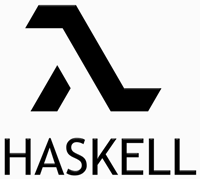Haskell is an advanced functional programming language, providing easy integration with other languages, built-in concurrency, and rich libraries.  Haskell programming is centered on evaluating expressions rather than executing instructions.

2) Mention what are the benefits of Haskell expression?

• In Haskell, variable, data structure etc. is immutable
• Haskell expression has no issues like updating global variables or printing to the screen
• Everytime calling the same function with the same argument will result in the same output
• It is possible to decouple I/O from the rest of the code, reducing programming error; it is very important feature of Haskell programming

A monad in Haskell is just a type for which the >>= operation is defined. Haskell’s I/O is based on Monads. It’s a specific way of binding operations together or in other words, it’s a way of wrapping things and provide a method to perform operations on the wrapped stuff without unwrapping it.

Each monad has its own application of the bind function like

5) Explain the type system for Haskell?

• While working with Haskell, the first step involves in writing a Haskell program is usually to write down all the types.
• Haskell language is like a transcript just by looking at the function’s type it will tell you about what the function might do
• Turns run-time errors into compile time errors, it is better to fix errors up front6) Explain how function is defined in Haskell?

Function definition in Haskell consists of a number of condition equations.  At the beginning of each, after the function name, there are patterns that show to which data each equation applies. After that there are multiple clauses, representing different cases and a where clause to hold local definitions.

7) Explain what is the difference between \$ (dollar sign) and . (dot) ?

In Haskell, \$ sign operator is used to avoid parenthesis, anything that appears after it will take precedence over anything that comes before. For example, (putStrLn .show) (1+1) can be replaced by putStrLn . show \$ 1+1.  While,. (dot) primary function is to chain function and not to avoid parenthesis.

8) Mention what is the difference between Haskell and Erlang?

 Haskell Erlang It caters features like higher order functions, equations, lazy evaluation, pattern matching over algebraic data type, etc. Haskell program is a collection of modules consists of values, data types, type synonyms, etc. A Haskell module imports definitions from other modules and re-export some of them including some of its own definition making them available to other modules. There is no built in support for concurrency in Haskell Haskell features static typing In some Haskell refractoring, type information is needed in order to succeed _________________ Haskell is more useful for complex and symbolic computation Erlang offers features like pattern matching, higher order functions, concurrency, dynamic code reloading, fault tolerance, etc. In Erlang, a module can only export functions, which are defined in the module itself. Erlang has built in support for concurrency Erlang features dynamic typing For most Erlang refractoring, type information is needed Erlang’s elementary data types are numbers, atoms, process identifiers, binaries and ports Erlang excels at doing simple tasks with high concurrency

9) Explain why Haskell algebraic data types are closed?

Haskell algebraic data types are closed because it makes it a lot easier to write total functions.  Functions that produce a result, for all possible values of its type.

10) Explain what is Prelude in Haskell?

In Haskell, prelude is a module that consists of a bunch of standard definitions that gets implicitly imported into Haskell program.

11) List out the numeric types in the Haskell “prelude”?

In Haskell, there are five numeric types that includes

• Int: It is an integer having at least 30 bits of precision
• Integer: It is an integer having unlimited precision
• Float: It is a single precision floating point number
• Double: It is a double point precision floating point number
• Rational: It is a fraction type with no rounding error

12) Mention how data types are combined in Haskell?

In Haskell, data types are combined in two ways

• List: It goes in [square brackets]
• Tuples: It goes in (parenthesis)

13) Mention what are the types of polymorphism you will encounter in Haskell?

In Haskell, there are two types of polymorphism

• Parametric Polymorphism: A function is parametrically polymorphic, if it behaves equally for all types, in at least one of its type parameters
• Bounded Polymorphism: You have bounded polymorphism or ad hoc, if you have custom behavior that you want to have for certain set of types

14) Explain how you can implement “ord” for algebraic data types in Haskell?

In Haskell, the best way to implement “ord” is just to add deriving (Eq, Ord) to the type’s definition.

15) Explain why “lazy evaluation” in Haskell is useful?

In Hazkel, lazy evaluation is useful due to following reasons

• Values will not be computed if they are not going to be used
• Haskell makes sure that the order in which the expressions are evaluated will never affect their result.
• Also, it allows the infinite lists

16) Explain what is the difference between “data” and “New type” in Haskell?

• Newtype: It guarantees that your data will have exactly the same representation at runtime, like the type that you wrap
• Data: It declares a brand new data structure at runtime

17) Mention what is the difference between Haskell (++) and (:)?

• (:) operator: It is known as the “cons” operator and is used to append a head element to a list
• (++) operator: It is a list concatenation operator, and it takes two operands and combine them into a single list
0 replies